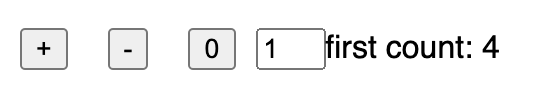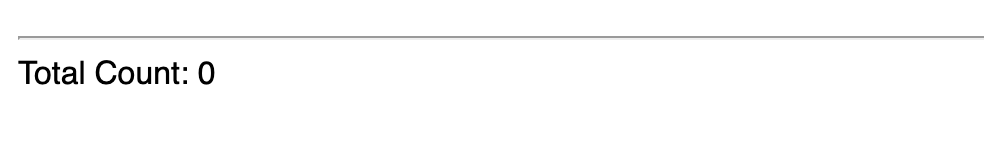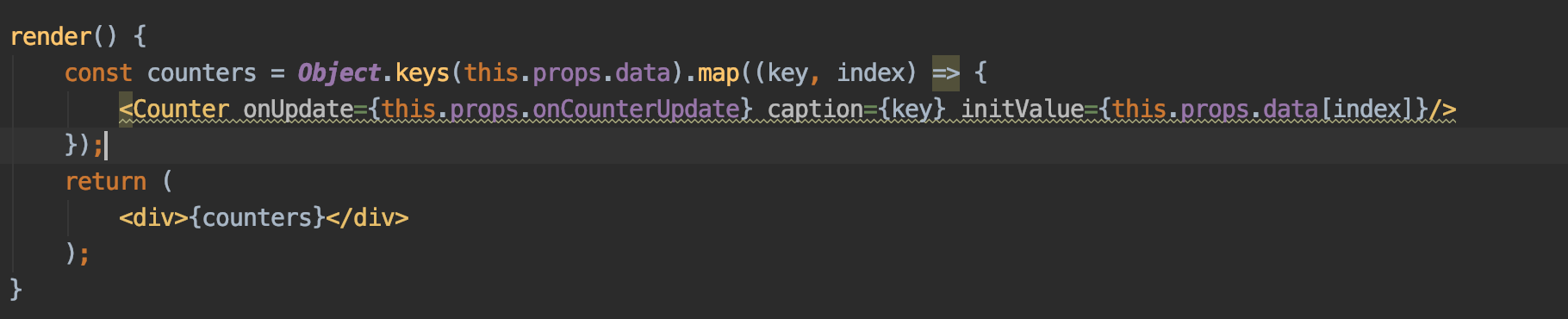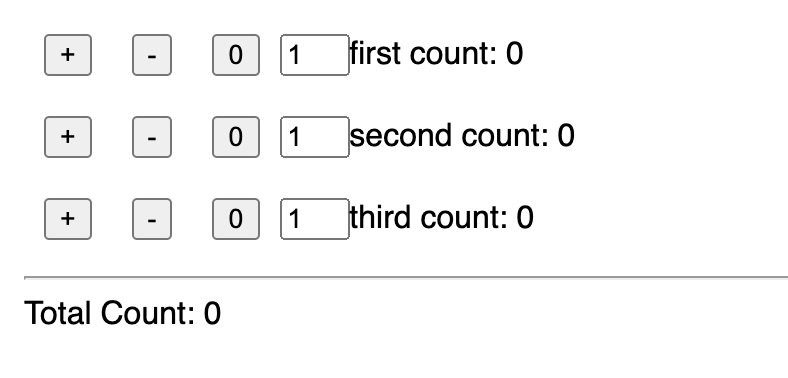### 1. Purpose

In this post, I would demo how to solve the javascript arrow function not working problem when developing a react component.

### 2. The problem and the solution

#### 2.1 The problem

I am trying to make a repeatable component in react application. Let’s say there is a component named `Counter`, here is the UI of it:Now I want to make a CounterList, which is composed of many counters,here is the code:

``````class CounterList extends Component {
constructor(props) {
super(props);
}

render() {
//this.props.data is an object
const counters = Object.keys(this.props.data).map((key, index) => {
<Counter />
});
return (
<div>{counters}</div>
);
}
}
``````

Instead of list of counters, we got nothing:And there are warning on the code:Why?

#### 2.2 The solution

The code that is working should be as follows:

``````        const counters = Object.keys(this.props.data).map((key, index) => (
<Counter onUpdate={this.props.onCounterUpdate} caption={key} initValue={this.props.data[index]}/>
));
``````

Now if the data is:

``````this.initMap = {"first":0,"second":10,"third":20};
``````

then the UI is:### 3. Summary

In this post, I demonstrated how to solve the problem when using arrow function to return objects, the key point is to use the parentheses instead of curly braces. That’s it, thanks for your reading.